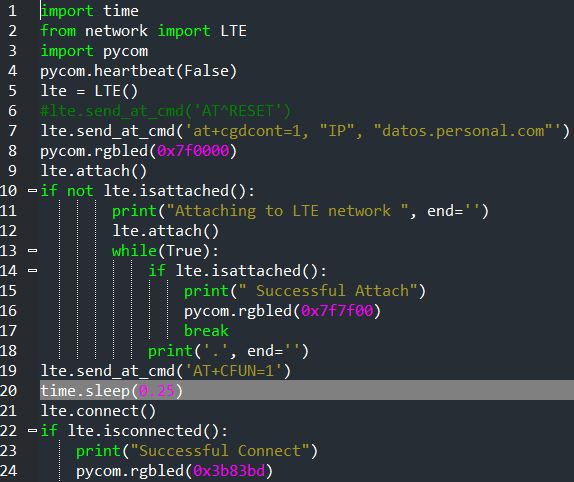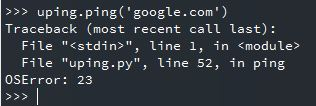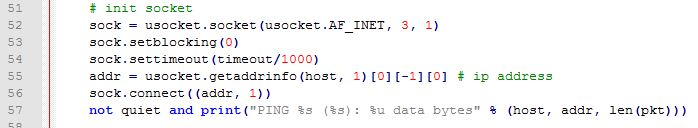# uping doesn´t work on LTE-M1

• I am attached and connected to LTE-M in Argentina :
lte.send_at_cmd('at+cgdcont=1, "IP", "datos.personal.com"')
Also enable the functionality +CFUN=1When I import uping.py file and try uping.ping('google.com') I got an error in line 52I thinks it is a modem configuration issue, something I have to define as I was playing with different at commands after lte.pppsuspend() , and one time when I return to LTE lte.pppresume() , it worked , but I didn´t save the configuration and since then I can not make it run.
Any idea to configure in the modem or in the uping script ?[uping.py]

Also I can not run the simple LTE example

s = socket.socket()
s = ssl.wrap_socket(s)
s.send(b"GET / HTTP/1.0\r\n\r\n")
print(s.recv(4096))

• @kjm thanks for your reply , the problem was the pppsuspend/resume ,absolutely it hangs the modem for internet. The uping file and the ping file you have sent works properly.

1. I've found pppsuspend/resume to be unreliable, maybe avoid them for the time being & use lte.disconnect() instead if you want to run an at cmd
2. Try to limit yourself to one query per post, I've found it improves your chances of getting a reply if you don't conflate multiple issues.
3. There are multiple versions of most micropython libraries, many of them not functional on pycom product. Try running the version of ping below on an attached/connected gpy
``````def checksum(data):
if len(data) & 0x1: # Odd number of bytes
data += b'\0'
cs = 0
for pos in range(0, len(data), 2):
b1 = data[pos]
b2 = data[pos + 1]
cs += (b1 << 8) + b2
while cs >= 0x10000:
cs = (cs & 0xffff) + (cs >> 16)
cs = ~cs & 0xffff
return cs

def ping(host, count=4, timeout=5000, interval=10, quiet=False, size=64):
import utime
import uselect
import uctypes
import usocket
import ustruct
import uos

# prepare packet
assert size >= 16, "pkt size too small"
pkt = b'Q'*size
pkt_desc = {
"type": uctypes.UINT8 | 0,
"code": uctypes.UINT8 | 1,
"checksum": uctypes.UINT16 | 2,
"id": (uctypes.ARRAY | 4, 2 | uctypes.UINT8),
"seq": uctypes.INT16 | 6,
"timestamp": uctypes.UINT64 | 8,
h.type = 8 # ICMP_ECHO_REQUEST
h.code = 0
h.checksum = 0
h.id[0:2] = uos.urandom(2)
h.seq = 1

# init socket
#sock = usocket.socket(usocket.AF_INET, usocket.SOCK_RAW, 1)
sock = usocket.socket(usocket.AF_INET, 3, 1)
sock.setblocking(0)
sock.settimeout(timeout/1000)
try:
except IndexError:
not quiet and print("Could not determine the address of", host)
return None
not quiet and print("PING %s (%s): %u data bytes" % (host, addr, len(pkt)))

seqs = list(range(1, count+1)) # [1,2,...,count]
c = 1
t = 0
n_trans = 0
n_recv = 0
finish = False
while t < timeout:
if t==interval and c<=count:
# send packet
h.checksum = 0
h.seq = c
h.timestamp = utime.ticks_us()
h.checksum = checksum(pkt)
if sock.send(pkt) == size:
n_trans += 1
t = 0 # reset timeout
else:
seqs.remove(c)
c += 1

# recv packet
while 1:
socks, _, _ = uselect.select([sock], [], [], 0)
if socks:
resp = socks.recv(4096)
resp_mv = memoryview(resp)
# TODO: validate checksum (optional)
seq = h2.seq
if h2.type==0 and h2.id==h.id and (seq in seqs): # 0: ICMP_ECHO_REPLY
#t_elasped = (utime.ticks_us()-h2.timestamp) / 1000
t_elasped = utime.ticks_diff(h2.timestamp, utime.ticks_us()) / 1000
ttl = ustruct.unpack('!B', resp_mv[8:9]) # time-to-live
n_recv += 1
not quiet and print("%u bytes from %s: icmp_seq=%u, ttl=%u, time=%f ms" % (len(resp), addr, seq, ttl, t_elasped))
seqs.remove(seq)
if len(seqs) == 0:
finish = True
break
else:
break

if finish:
break

utime.sleep_ms(1)
t += 1

# close
sock.close()
ret = (n_trans, n_recv)
not quiet and print("%u packets transmitted, %u packets received" % (n_trans, n_recv))
return (n_trans, n_recv)